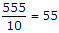# Electronics and Communication Engineering - Microprocessors

### Exercise :: Microprocessors - Section 4

26.

The number of logic or arithmetic operations with `ALU 1C 74181` can carry out is

 A. 4 B. 8 C. 16 D. 32

Explanation:

No answer description available for this question. Let us discuss.

27.

6 bytes means

 A. 6 bits B. 24 bits C. 48 bits D. 96 bits

Explanation:

One byte has 8 bits.

28.

In the integer expression `- A * B div C + D` which operation is done first in Pascal

 A. - B. * C. div D. +

Explanation:

In Pascal binary has highest priority.

29.

In Pascal if I = 55, 10 * I div 10 =

 A. 55 B. 50 C. 5.5 D. 5

Explanation:

In Pascal multiplication has higher precedence than division so 10 x 55 =.

30.

In FORTRAN 77 IFIX (-73.2) gives the value

 A. 73 B. -73 C. -7 D. -73.2